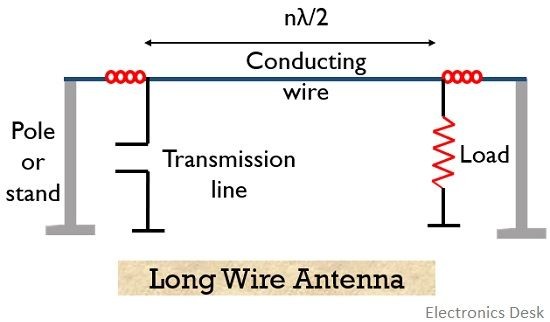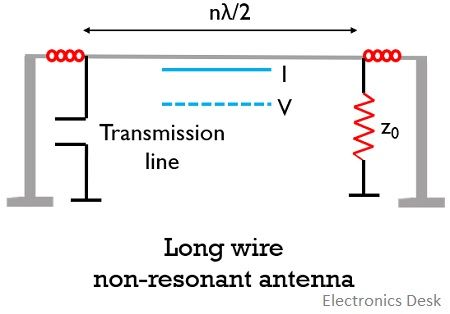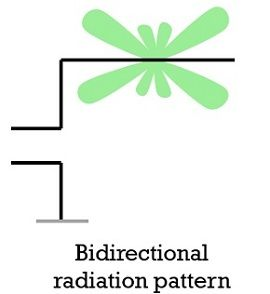# Long Wire Antenna

A long wire antenna is a type of antenna in which the length of the wire is usually greater than the length of the half-wave dipole i.e., λ/2. These are also known as harmonic antennas.

It offers operating frequency range between 500 kHz to 30 MHz.

## Content: Long Wire Antenna

### Introduction

We know that antennas generally have length λ/2 or λ/4 known as a half-wave dipole or quarter-wave monopole respectively. However, in order to have different types of antennas, the wires used are considered of arbitrary length.

So, when we have an antenna whose length is greater than λ/2, then such antennas are known as long wire antenna.

A noteworthy point over here is that the length of the long wire antenna is usually considered as multiple of half wavelength.

Thus, the length of the long wire antenna is given as

L = n λ/2

Long wire antennas are further classified as V antennas, inverted v antennas, rhombic antennas etc.

### Construction

Long wire antennas offer simplest constructional structure. This is so because here a long straight wire is considered whose length is a multiple of λ/2.

These can be horizontally or vertically placed. However, sometimes the orientation is sloppy with respect to the ground. The external excitation is provided through feed lines. And the feed line is provided at the centre, the end or somewhere in between the length of the wire.

It is to be noted that the orientation of the antenna with respect to the ground shows polarization of the antenna. And the position of the feed point represents the direction of the lobe.The ease of construction offered by these antennas is due to the fact that a simple conducting wire is end to end connected between a transmitting and receiving stations.

The direct connection of the long wire between the two ends allows it to accept the signal from the transmitting station and radiate the wave so that it can be received at the other end.

## Working of Long Wire Antenna

As the long wire antenna is said to be a combination of multiple half-wave dipoles; thus, its principle of operation is the same as the half-wave dipole antenna.

We know that these are the type of antennas whose length is greater than half wavelength. So, when such a long conducting wire is excited with the input voltage, then the charge carriers drift according to the applied half of the signal. This means if positive half of the signal is applied, then charge carriers experience attractive force while due to the negative half cycle, the charge carriers experience repulsion.

This cumulative action of the charge carriers within the conductor generates a varying electric field. Hence in this way the signal is radiated by the long wire antenna at the other end.

The long wire antenna is more than half wavelength long; however, greater the multiple of λ/2, more will be the directivity. Thus, with the increase in the length of the long wire antenna, the directivity also gets better.

It is noteworthy in case of a long wire antenna that for lowest frequency of operation the length is generally considered as half wavelength and external feeding is provided at the end.

Generally, it is placed in horizontal manner, with respect to ground thus radiates horizontally polarized wave at an angle between 17° to 24° relative to the surface of the earth.

Long wire antennas are mainly classified as:

• Long wire resonant antenna: It is a type of long wire antenna that offers unmatched load thus gives rise to standing waves.

Basically, the load of the resonant type of long wire antenna is unterminated. Due to unterminated load, standing waves exist along the length of the wire thus is bidirectional in nature.

The figure below represents the resonant type of antenna:Here the length of the antenna is directly proportional to the operating frequency.

• Long wire non-resonant antenna: This antenna is terminated properly with characteristic impedance. Thus, is non-resonating in nature hence called so.

Here standing waves do not exist; the reason behind this is that all the incident waves get collected at the terminated end. Thus, the reflected wave is absent here.

The figure below represents the non-resonant type of antenna:Therefore, the radiation pattern of this type of antenna is unidirectional, as waves follow only forward path. Unlike the resonant type of antenna, here the length of the wire is inversely proportional to the frequency.

The figure below represents the radiation pattern of long wire resonant antenna:Here the bidirectional nature of the radiation pattern shows the existence of standing waves.

Now see the radiation pattern of the non-resonant type of antenna:The unidirectional radiation pattern shows that due to the properly terminated load, standing waves do not exist in this type of antenna.

1. Long wire antennas are low-cost antennas.
2. It offers ease of construction.
3. The gain and directivity are improved with the use of a long wire antenna.
4. It offers multiband operation.